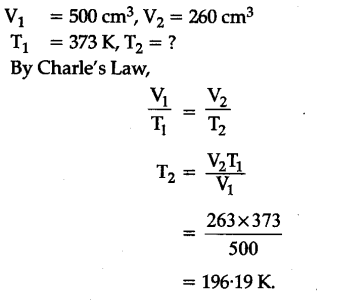# A sample of helium has a volume of 500 c\${{m}^{3}}\$ at 373 K. Calculate the temperature at which the volume will become 260 c\${{m}^{3}}\$. Assume that the pressure is constant